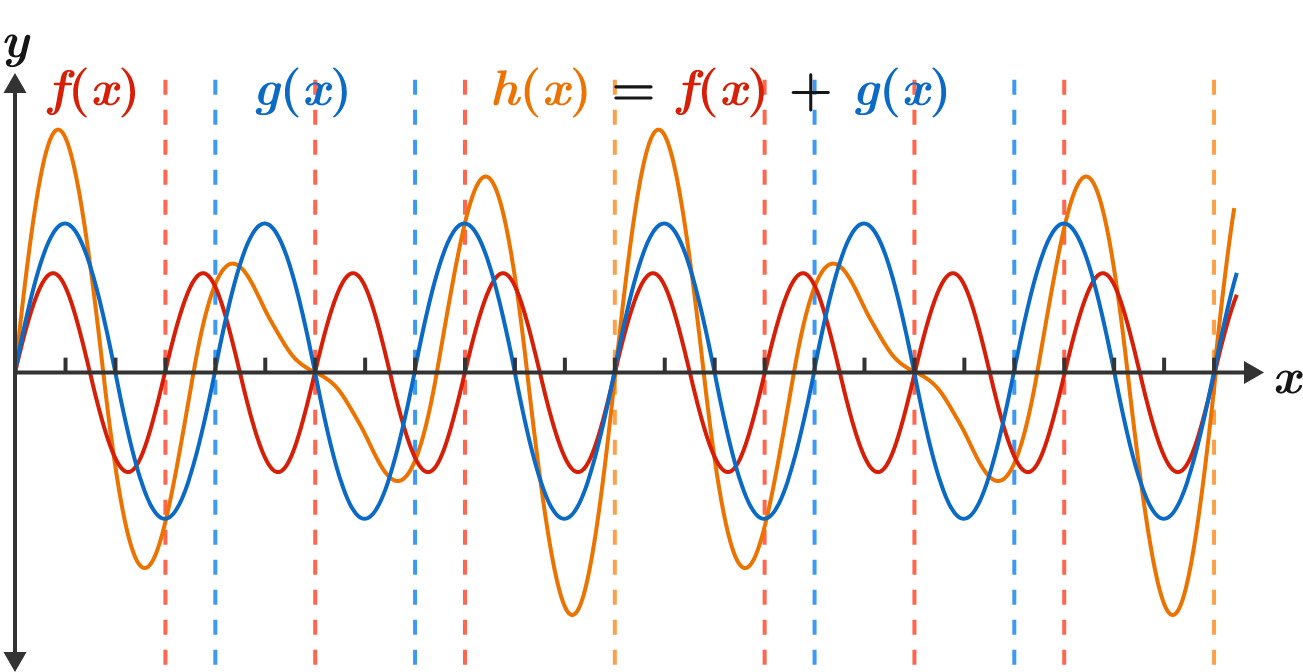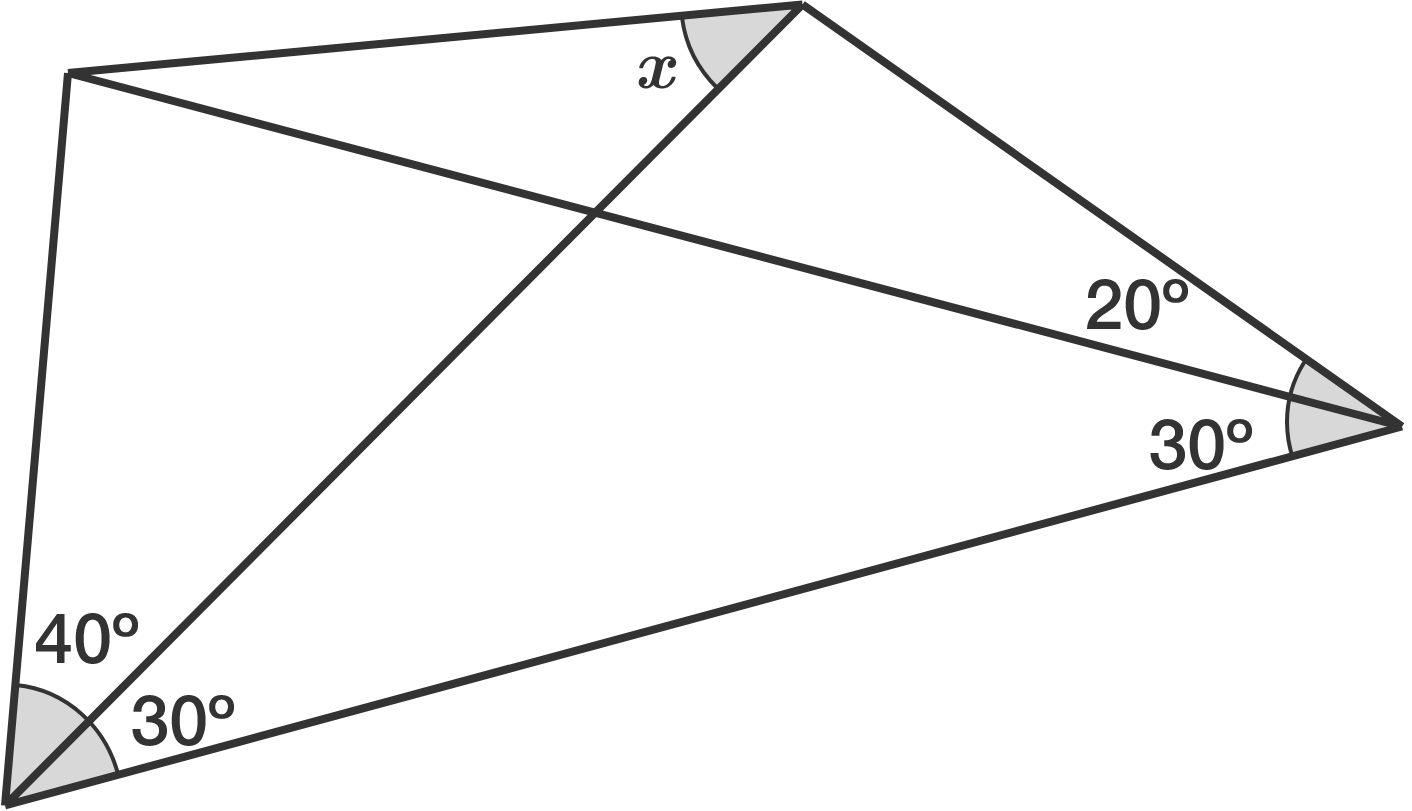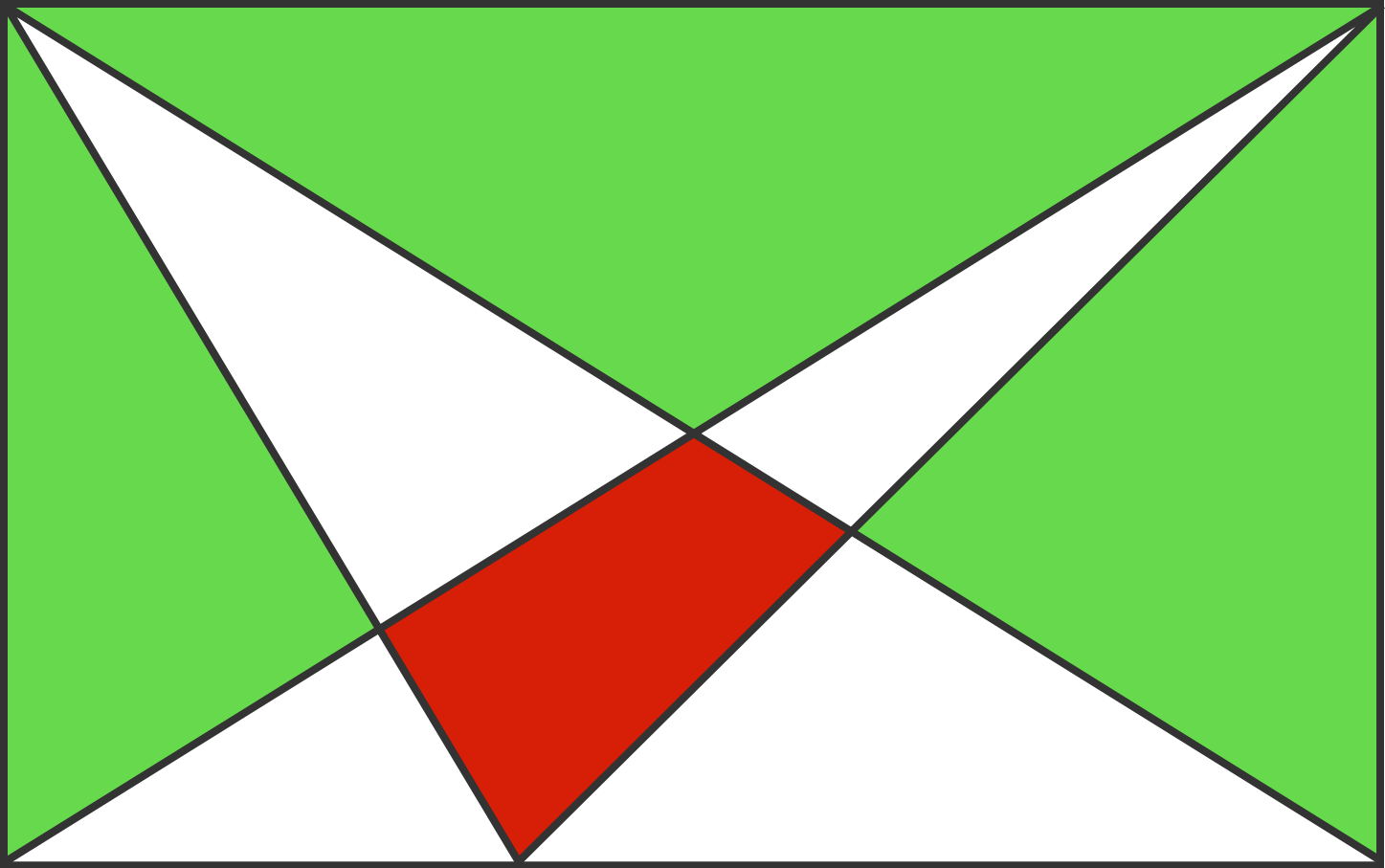# Problems of the Week

Contribute a problem

# 2018-06-25 Intermediate

$\color{#D61F06}f(x),$ $\color{#3D99F6}g(x),$ and the function ${\color{#EC7300}h(x)}={\color{#D61F06}f(x)}+{\color{#3D99F6}g(x)}$ have fundamental periods of $3,$ $4,$ and $\text{lcm}(3,4)=12,$ respectively.

If $p(x)$ and $q(x)$ have fundamental periods $2$ and $\pi,$ respectively, what is the fundamental period of $r(x)=p(x)+q(x)?$What is the measure of angle $x$ (in degrees)?Anna, Brenda, and Cynthia are taking turns playing a two-person game. Each game, the player with the least amount of money bets it all, and the opponent calls it. The results of the games are as follows:

• Anna and Brenda play the first game. Anna wins and thereby doubles her money.
• Brenda and Cynthia play the second game. Brenda wins and doubles her money.
• Anna and Cynthia play the third game. Cynthia wins and doubles her money.

All three of them end up with equal amounts of money.

They each started with an integer number of cents. One of the players started with 50 cents—who was it?The area of the whole rectangle is 160 and the area in red is 12.

What is the area in green?

Vision is enabled by light scattering off of an object and taking a straight line path directly to your eye. On a clear day, nearly all of the light from an object makes it to your eye (assuming no large objects block the path).But on a foggy day, the atmosphere is filled with droplets of water that redirect light in random directions if it hits them, blurring the image. As the fog gets thicker and thicker, faraway images become badly scrambled.

Suppose that on a foggy day there are roughly $N_\text{droplet} = 200$ water droplets per cubic centimeter of atmosphere, and that each droplet is about $d_\text{droplet} = \SI{20}{\micro\meter}$ in diameter. About how far away can an object be located before $90\%$ of its image is scrambled by the fog?

Assume that when light scatters off of a water droplet, it goes off in a random direction.

×

Problem Loading...

Note Loading...

Set Loading...